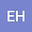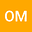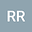MULTIPLICITY OF SOLUTIONS TO CLASS OF NONLOCAL ELLIPTIC PROBLEMS WITH CRITICAL EXPONENTS
•••OLIMPIO MIYAGAKI
Universidade Federal de São Carlos Centro de Ciências Exatas e de Tecnologia
Author ProfileRodrigo Rodrigues
Author Profile## Abstract

In this paper, we establish existence of infinitely many weak solutions for a class of quasilinear stationary Kirchhoff type equations, which involves a general variable exponent elliptic operator with critical growth. Precisely, we study the following nonlocal problem \begin{equation*} \begin{cases} -\displaystyle{M}(\mathscr{A}(u))\operatorname{div}\Bigl(a(|\nabla u|^{p(x)})|\nabla u|^{p(x)-2}\nabla u\Bigl) = \lambda f(x,u)+ |u|^{s(x)-2}u \text{ in }\Omega, \\ u = 0 \text{ on } \partial \Omega, \end{cases} \end{equation*} where $\Omega$ is a bounded smooth domain of $\mathbb{R}^N,$ with homogeneous Dirichlet boundary conditions on $\partial \Omega,$ the nonlinearity $f:\overline{\Omega}\times \mathbb{R}\to \mathbb{R}$ is a continuous function, $a:\mathbb{R}^{+}\to\mathbb{R}^{+}$ is a function of the class $C^{1},$ $M:\mathbb{R}^{+}_{0}\to\mathbb{R}^{+}$ is a continuous function, whose properties will be introduced later, $\lambda$ is a positive parameter and $p,s\in C(\overline{\Omega})$. We assume that $\mathscr{C}=\{x\in \Omega: s(x)=\gamma^{*}(x)\}\neq \emptyset,$ where $\gamma^{*}(x)=N\gamma(x)/(N-\gamma(x))$ is the critical Sobolev exponent. We will prove that the problem has infinitely many solutions and also we obtain the asymptotic behavior of the solution as $\lambda\to 0^{+}$. Furthermore, we emphasize that a difference with previous researches is that the conditions on $a(\cdot)$ are general overall enough to incorporate some interesting differential operators. Our work covers a feature of the Kirchhoff’s problems, that is, the fact that the Kirchhoff’s function $M$ in zero is different from zero, it also covers a wide class of nonlocal problems for $p(x)>1,$ for all $x\in \overline{\Omega}.$ The main tool to find critical points of the Euler Lagrange functional associated with this problem is through a suitable truncation argument, concentration-compactness principle for variable exponent found in \cite{bonder}, and the genus theory introduced by Krasnoselskii.

#### Peer review status:ACCEPTED

21 Jul 2021Submitted to Mathematical Methods in the Applied Sciences
23 Jul 2021Submission Checks Completed
23 Jul 2021Assigned to Editor
30 Jul 2021Reviewer(s) Assigned
28 Oct 2021Review(s) Completed, Editorial Evaluation Pending
28 Oct 2021Editorial Decision: Revise Major